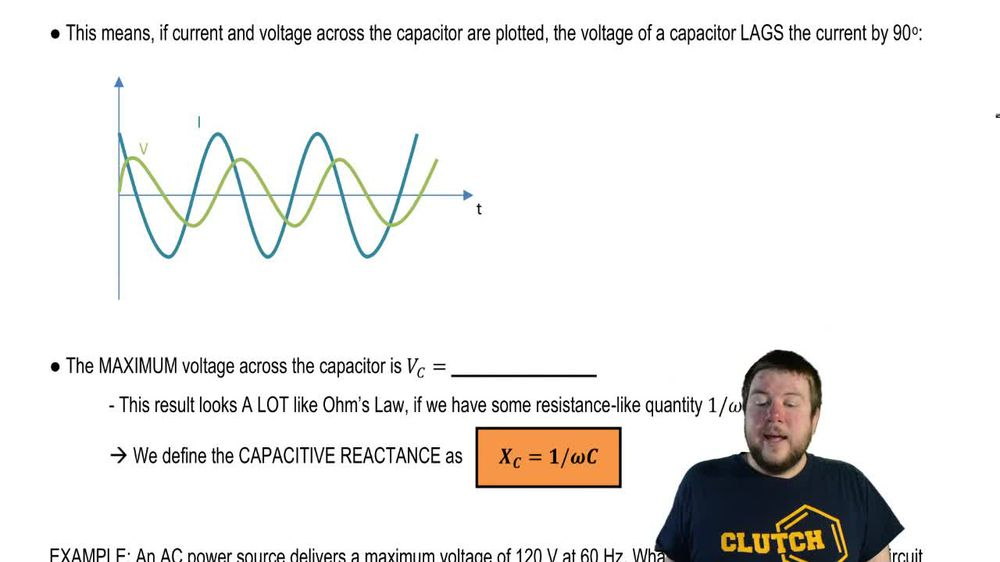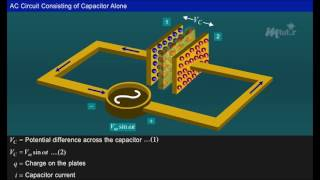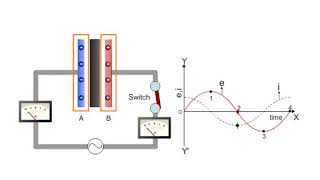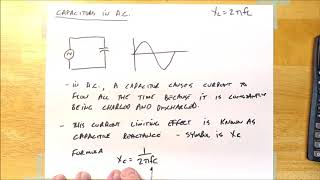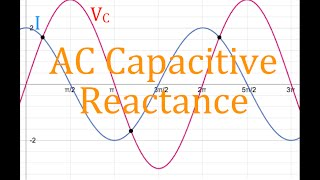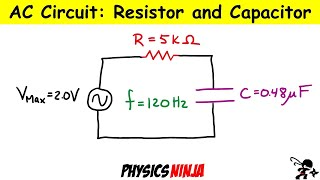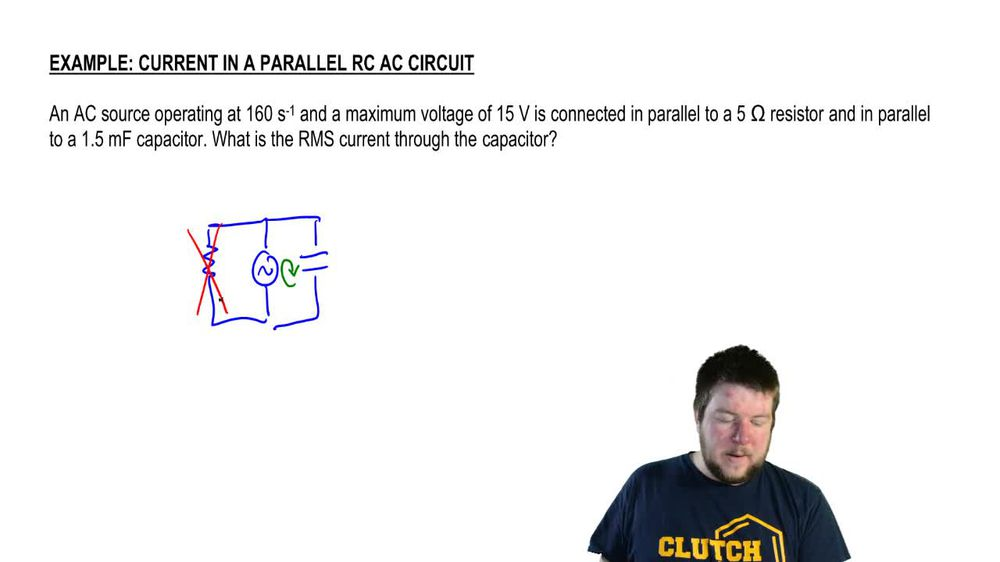Start typing, then use the up and down arrows to select an option from the list.
1. 31. Alternating Current2. Capacitors in AC Circuits# Current in a Parallel RC AC Circuit

by Patrick Ford
61 views
3
0
Hey, guys, let's do an example involving capacitors in a sea circuits and a C source operating at 160 inverse seconds. And at a maximum voltage of 15 volts is connected in parallel toe a five ohm resistor and in parallel toe, 1.5 million Farah capacitor. What does the RMS current through the capacitor? So what does this look like? Well, let's say we have an a c source. It's connected in parallel to a resistor, which we're told is that five home resistor and it's connected in parallel to a capacitor. And we want to know what is the RMS current through the capacitor. Okay, well, we can actually solve this problem without involving the resistor at all, because this forms its own loop, which means that kerchiefs loop rule applies to it. So whatever voltage the battery has, the capacitor has the same voltage, and we can completely ignore the resistor because it doesn't belong in this loop. Okay, so everything that happens is determined on li buy things within that loop. Okay, so the resistor doesn't actually affect this problem at all. It's just throwing at you to confuse you. Okay? Now we want to find the R. M s current. In order to know any RMS value, you have to know the maximum value, right? The R. M s current is going to be the maximum currents divided by the square root of two. Okay, so before we can know the r. M s, we have to know the maximum. Now, the maximum current through a capacitor is always going to be that maximum voltage across capacitor divided by the capacitive reactions. So the first thing we need to figure out is what is that capacitive reactant. It's just one over Omega Psi. What is Omega? We're told that it operates at 160 in for seconds. If this was hurts, we would assume that that was a linear frequency. But because the units are in for seconds, we're gonna assume that that is an angular frequency, so we can plug that right into here. 1 60. The capacitance is 1.5 million fare ads or 0.15 fair ads, and that whole thing comes out to 4.2 owns. Now that we know the capacitive reactant, we confined the maximum current through the capacitor. Okay, that maximum current is just going to be the maximum voltage across the capacitor, divided by that reactant. Now, what is the maximum voltage across the capacitor? Since it's in parallel with the source, it's just gonna be the maximum voltage of the source or volts. So there's gonna be 15 volts divided by 4.2 owns, which is 3.57 amps. Okay, so we know that maximum current all we need to do now is divided by the square root of two to figure out what the R. M s current is. So finally, the arm s current is 357 and divided by the square root of two, which is just 252 APS. Okay, And that is the answer to the question. All right, guys, Thanks for watching